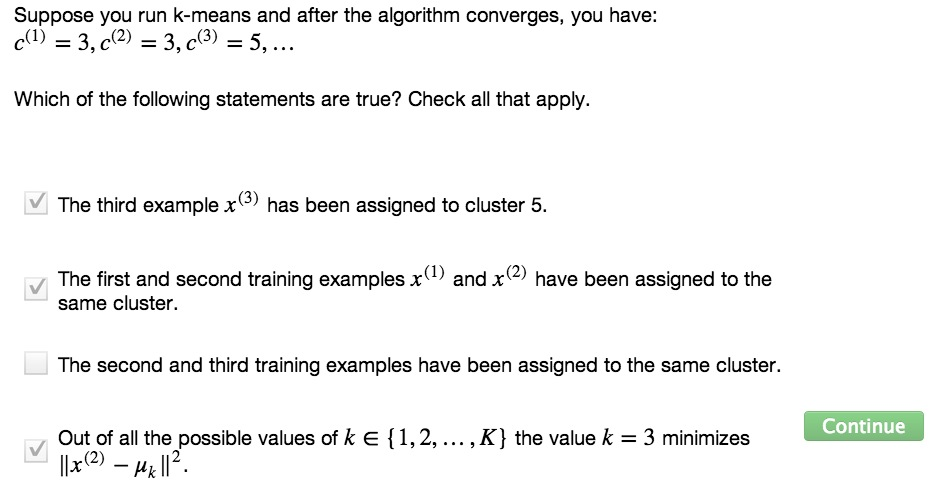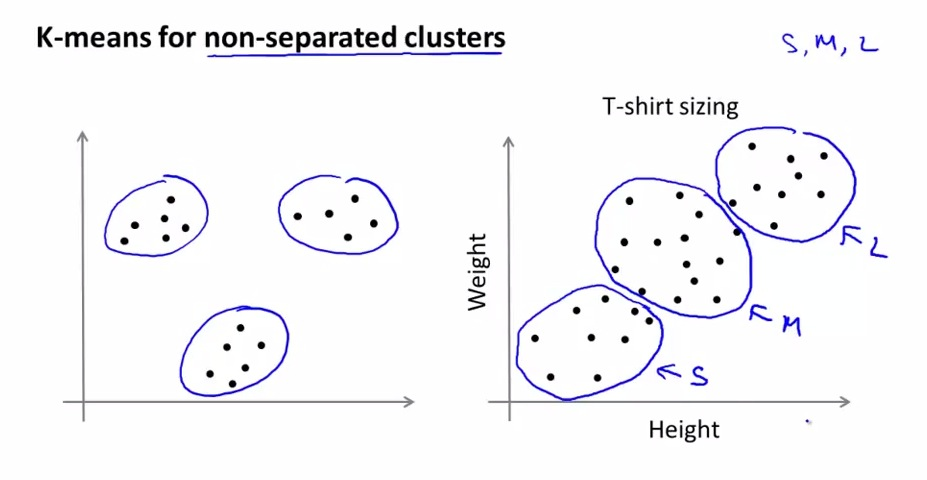• Clustering Algorithm is used to classify the data structure that not labeled at the beginning.
• K-means is one example of Clustering, and one of the most powerful and famous example of Clustering Algorithm to date.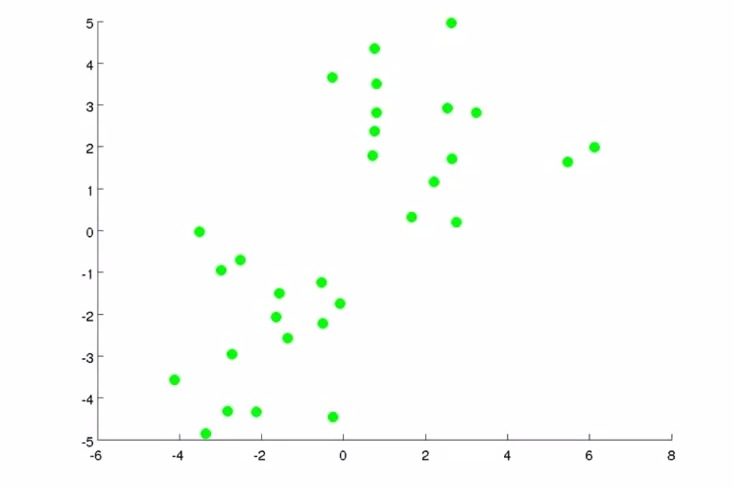• Two step in K-means
• Cluster Assignment Step
• Move Centroid Step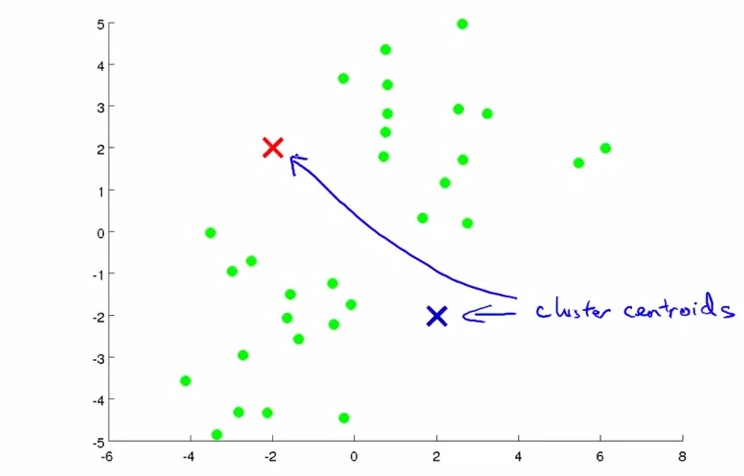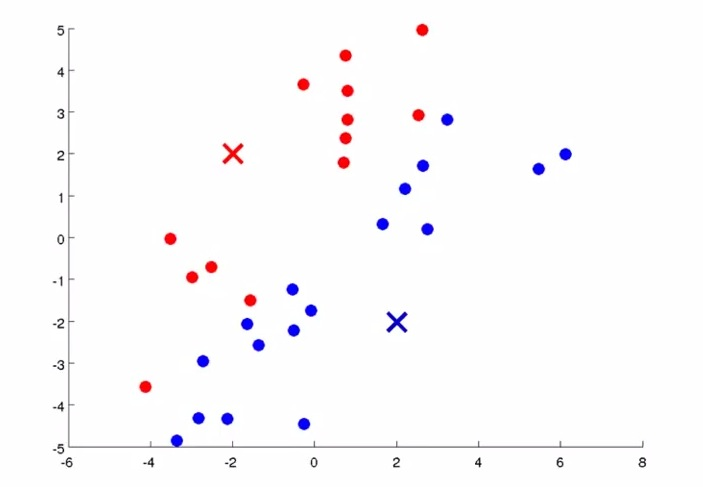• First step(Cluster Assignment Step), build n number of cluster centroids(number of cluster that we want)
• Color which one is closer between cluster centroids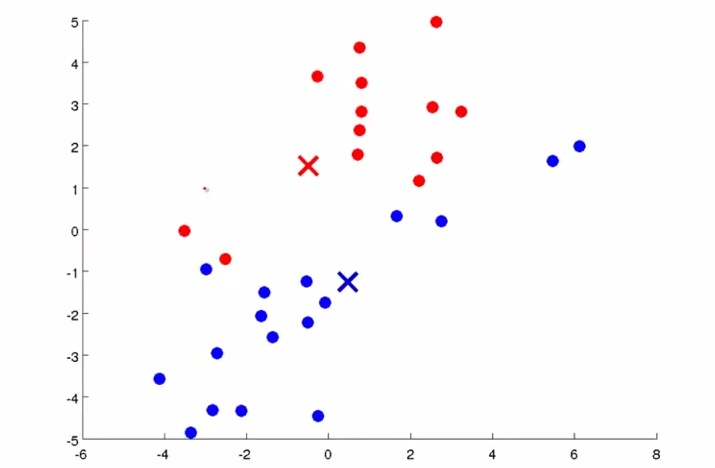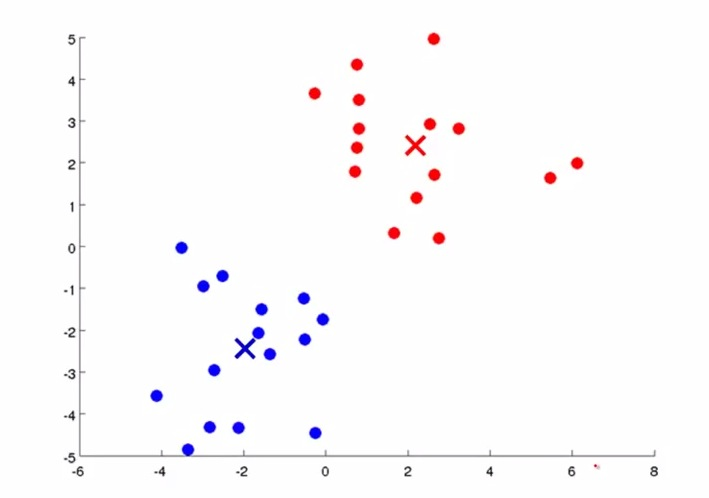• Second step(Move Centroids Step), take average/mean of the distance of all the examples that has the same color as the centroid has, and move it to the result point
• Go back to Cluster Assignment Step, and recolor it once again
• Again, continue back to Move Centroid Step,
• Do it again to Cluster Assignment Step
• Iterate it over and over again until the cluster centroids don't move any further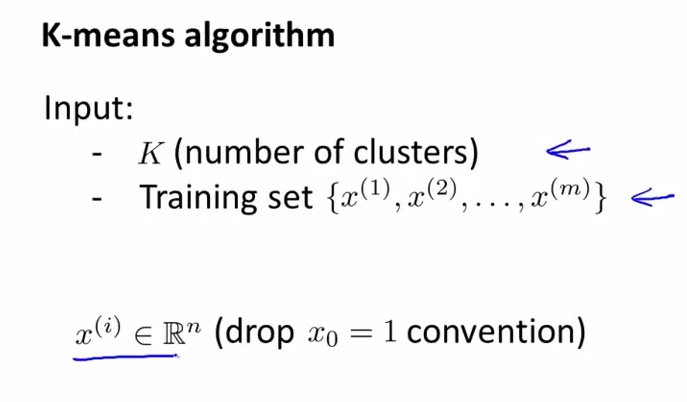• These are the mathematical step of K-means algorithm
• First let manually set K
• Remember that y value is not needed anymore
• without x0 convention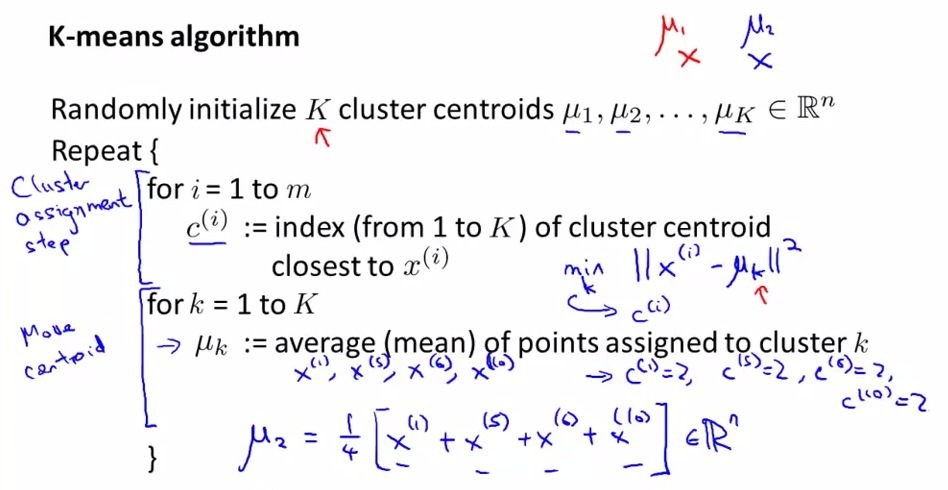• First initialize all the cluster randomly
• Then create some vector represent examples that the ith element is assigned with the index number of cluster that closest to it.(find the shortest length)
• Then for every cluster, get all the vector assigned earlier, take average distance(mean) produced midpoint among same index, and move the cluster relative to the index
• upper K denotes max number of K
• lower k is index of cluster centroids

• x is m-dimensional vector
• if sometimes there's one cluster centroid that has no point, best to eliminate it. Then it resulting to K-1 init, or reiinitialized it, although the first one is more wisely